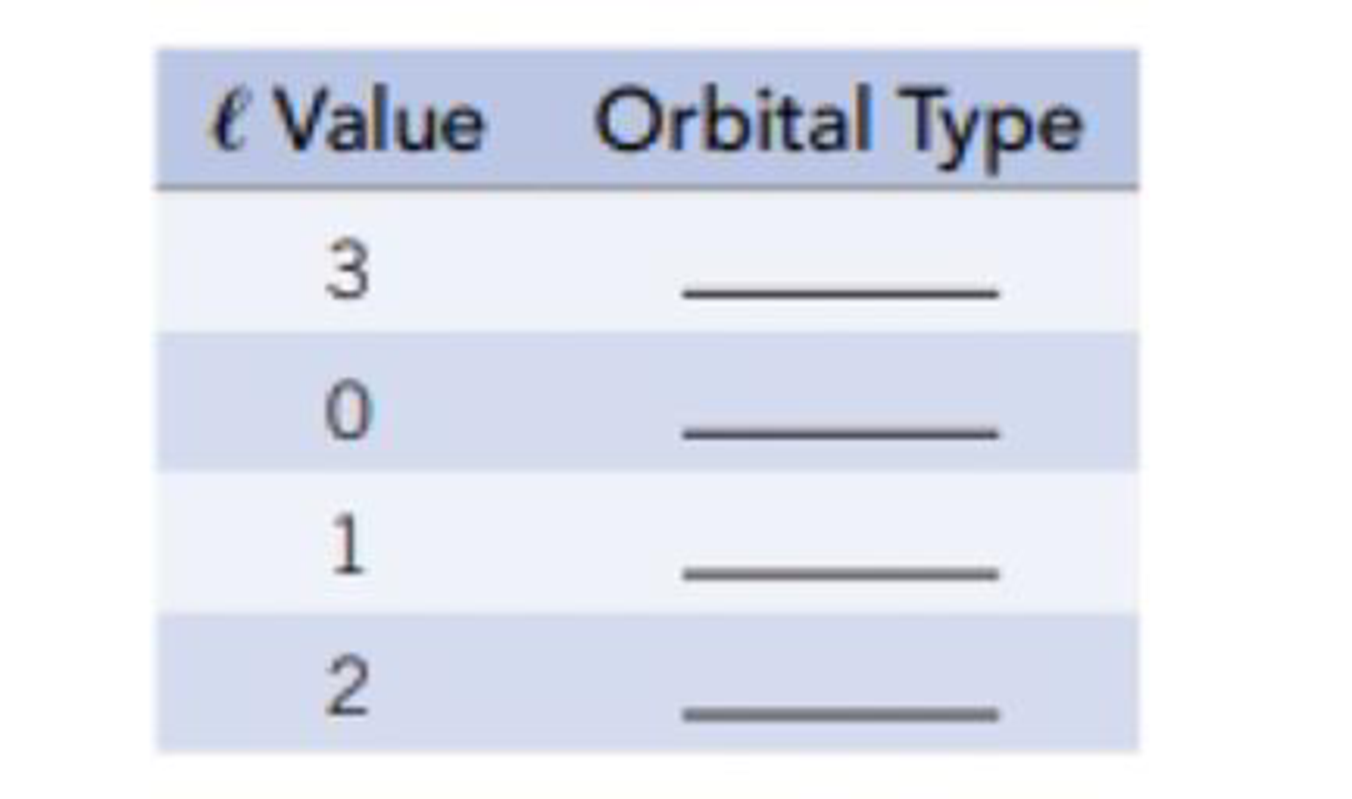Chapter 6, Problem 51GQ

Chapter
Section
Textbook Problem

Match the values of l shown in the table with orbital type (s, p, d, or f).Interpretation Introduction

Interpretation: The orbitals for the given values of l should be identified.

Concept introduction:

Quantum numbers are numbers, which explains the existence and the behavior of electron in an atom.

1. a) Principle quantum number is represented by n and this number describes the energy of the orbital and the size of an atom.
2. b) Angular momentum quantum number (or azimuthal quantum number) is represented by l and this number indicates the shape of the orbitals.
3. c) Magnetic quantum number is represented by ml and this number indicates the orientation of the orbital.
4. d) Spin quantum number is represented by ms and this number indicates the spin of the electron.

Angular momentum quantum number (or azimuthal quantum number) is represented by l and this number indicates the shape of the orbitals. Each value of l indicates subshell.

Explanation

Angular momentum quantum number (or azimuthal quantum number) is represented by l and this number indicates the shape of the orbitals.

The values of l when the principal quantum number is n are from 0 to (n1). Each value of l indicates subshell.

Therefore for l=0,1,2 and 3 represents s, p, d and f subshells For p subshells, l=1

Still sussing out bartleby?

Check out a sample textbook solution.

See a sample solution

The Solution to Your Study Problems

Bartleby provides explanations to thousands of textbook problems written by our experts, many with advanced degrees!

Get Started

According to genomic researchers, a single inherited gene is the probable cause of common obesity. T F

Nutrition: Concepts and Controversies - Standalone book (MindTap Course List)

In which domain and kingdom are humans classified?

Biology: The Dynamic Science (MindTap Course List)

What is the difference between type I and type II supernovae?

Horizons: Exploring the Universe (MindTap Course List)

Tell how you would try to prove to a doubter that air is matter.

Chemistry for Today: General, Organic, and Biochemistry

How does metabolic rate vary with temperature?

Oceanography: An Invitation To Marine Science, Loose-leaf Versin

Bacteria, Archaea, and Eukarya are three ________.

Biology: The Unity and Diversity of Life (MindTap Course List)